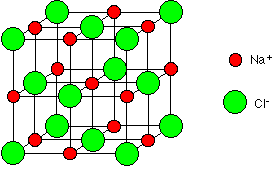# Question b2b2b

Mar 5, 2015

$\text{XY}$ will have a lattice energy of $\text{-2980 kJ/mol}$.

This is a very simple problem if you're familiar with the Born-Lande equation for calculating lattice energies. Here's how that looks likeI won't list what all the terms in this equation mean because all but three of them are not important. The three terms that you should focus on are

${z}^{+}$ $\to$ the charge on the positive ion;
${z}^{-}$ $\to$ the charge on the negative ion;
${r}_{0}$ $\to$ the distance between the two opposite ions;

Now, I believe that you are supposed to ignore the terms that are specific to individual ionic compounds, and assume that all the constants for your hypothetical salt are identical to those for CsF".

If that's the case, you can rewrite the above equation like this

$\text{E} = \frac{- {N}_{A} \cdot M \cdot {e}^{2}}{4 \cdot \pi \cdot {\epsilon}_{0}} \cdot \left(1 - \frac{1}{n}\right) \cdot \frac{| z {|}^{+} \cdot | z {|}^{-}}{r} _ 0$

$E = \text{CONSTANT} \cdot \frac{| z {|}^{+} \cdot | z {|}^{-}}{r} _ 0$

Now, because X"^(2+) has the same radius as Cs"^(+) and ${\text{Y}}^{2 -}$ has the sameradius as ${\text{F}}^{-}$, the term ${r}_{0}$ will be the same for both salts. Therefore,

$E = \text{CONSTANT} \cdot \left(| z {|}^{+} \cdot | z {|}^{-}\right)$

In the case of $C s F$, ${z}^{+}$ is +1 and ${z}^{-}$ is -1; you can use this to write the lattice energy of $\text{XY}$ using the lattice energy of $C s F$

${E}_{\text{XY") = E_("CsF}} \cdot \left(| z {|}^{+} \cdot | z {|}^{-}\right)$

Since $\text{XY}$ has ${z}^{+}$ equal to +2 and ${z}^{-}$ equal to -2, you'll get

${E}_{\text{XY") = E_("CsF") * (|+2| * |-2|) = 4 * E_("CsF}}$

E_("XY") = 4 * ("-744 kJ/mol") = "-2976 kJ/mol"

Rounded to three sig figs, the answer will be

E_("XY") = "-2980 kJ/mol"#

Mar 5, 2015

The lattice energy of $X Y$ is $- 2976 \text{kJ/mol}$

If we consider 2 charges ${q}_{1}$ and ${q}_{2}$ separated by a distance $r$ they are attracted by a force which is proportional to the product of the charges and inversely proportional to the square of the distance between them:

$F \propto \frac{{q}_{1} {q}_{2}}{{r}^{2}}$

So $F = k \frac{{q}_{1} {q}_{2}}{{r}^{2}}$

We can find the work done in separating these charges from a distance $r$ to infinity by integrating between these limits:

$W = k {\int}_{r}^{\infty} \frac{{q}_{1} {q}_{2}}{{r}^{2}} . \mathrm{dr}$

From which we get:

$W = - k \frac{{q}_{1} {q}_{2}}{r}$

This means that if we double the charges the work done required to separate them would go up by 2 x 2 = 4.

This means that in your example the lattice energy would increase to 4 x -744 = $2976 \text{kJ/mol}$, provided $r$ does not change.

In this answer I have only considered 2 charges separated by a distance $r$. In reality ionic crystals are made up of giant lattices with attractive and repulsive forces moving out in 3 dimensions and decreasing with distance.A more accurate expression for lattice enthalpy $U$ which accounts for this is given by:

$U = - \frac{N M {z}^{2} {e}^{2}}{4 \pi {\epsilon}_{0} {r}_{0}} \left(1 - \frac{1}{n}\right)$

I won't go further into the details of this. If you want you can look up "Madelung Constant".